Bingham Distribution edit page

## Theory

The Bingham distribution has the density function

$f(g;K,U) = _1\!F_1 \left(\frac{1}{2},2,K \right)^{-1} \exp \left\{ g^T UKU g \right\},\qquad g\in S^3,$

where $$U$$ is an $$4 \times 4$$ orthogonal matrix with unit quaternions $$u_{1,..,4}\in S^3$$ in the columns and $$K$$ is a $$4 \times 4$$ diagonal matrix with the entries $$k_1,..,k_4$$ describing the shape of the distribution. $$_1F_1(\cdot,\cdot,\cdot)$$ is the hypergeometric function with matrix argument normalizing the density.

The shape parameters $$k_1 \ge k_2 \ge k_3 \ge k_4$$ give

• a bipolar distribution, if $$k_1 + k_4 > k_2 + k_3$$,
• a circular distribution, if $$k_1 + k_4 = k_2 + k_3$$,
• a spherical distribution, if $$k_1 + k_4 < k_2 + k_3$$,
• a uniform distribution, if $$k_1 = k_2 = k_3 = k_4$$,

The general setup of the Bingham distribution in MTEX is done as follows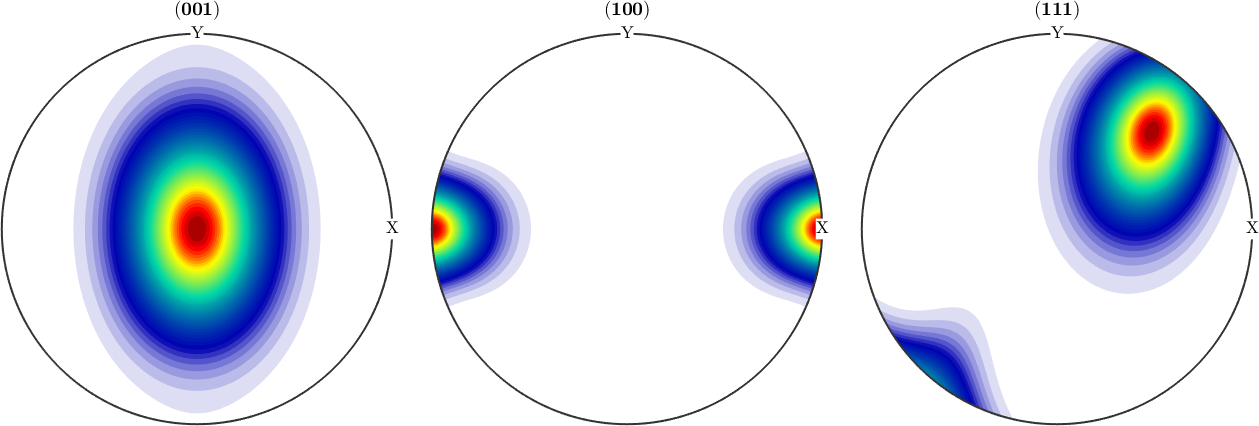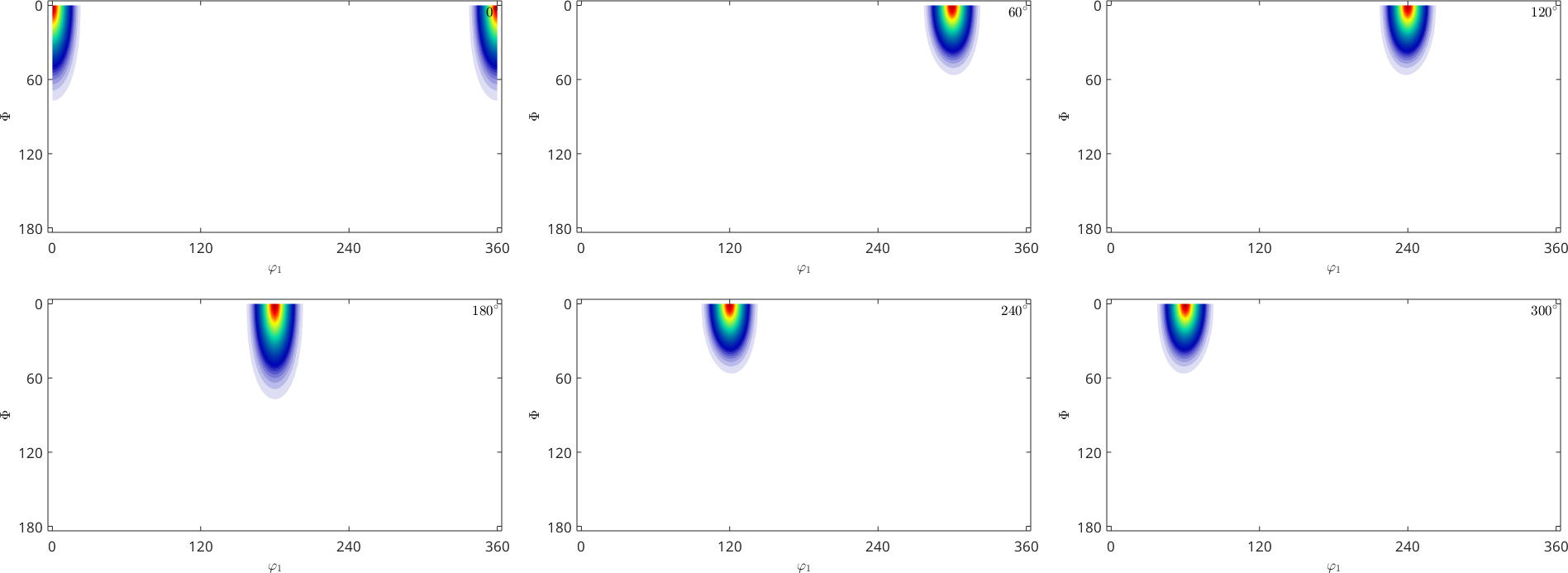## The bipolar case and unimodal distribution

First, we define some unimodal odf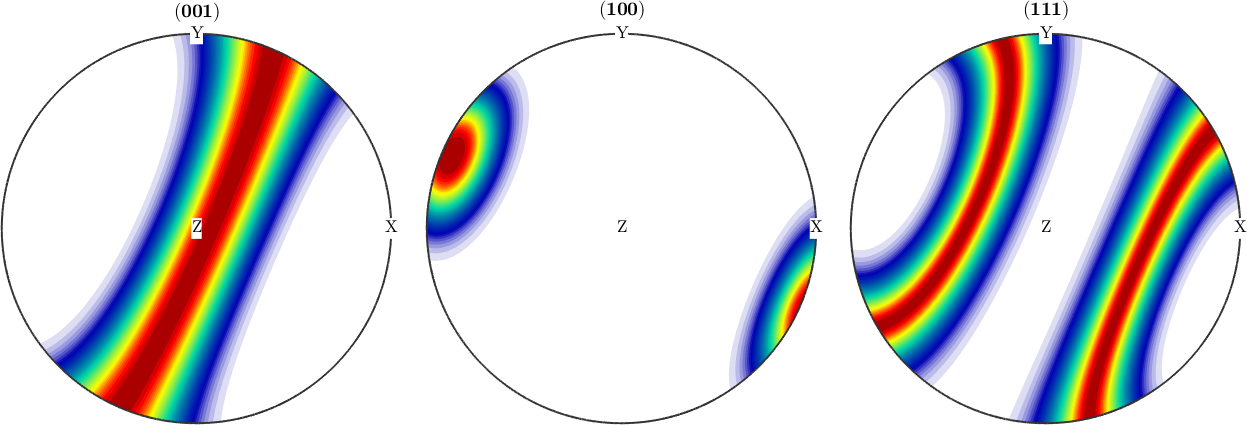Next, we simulate individual orientations from this odf, in a scattered axis/angle plot in which the simulated data looks like a sphere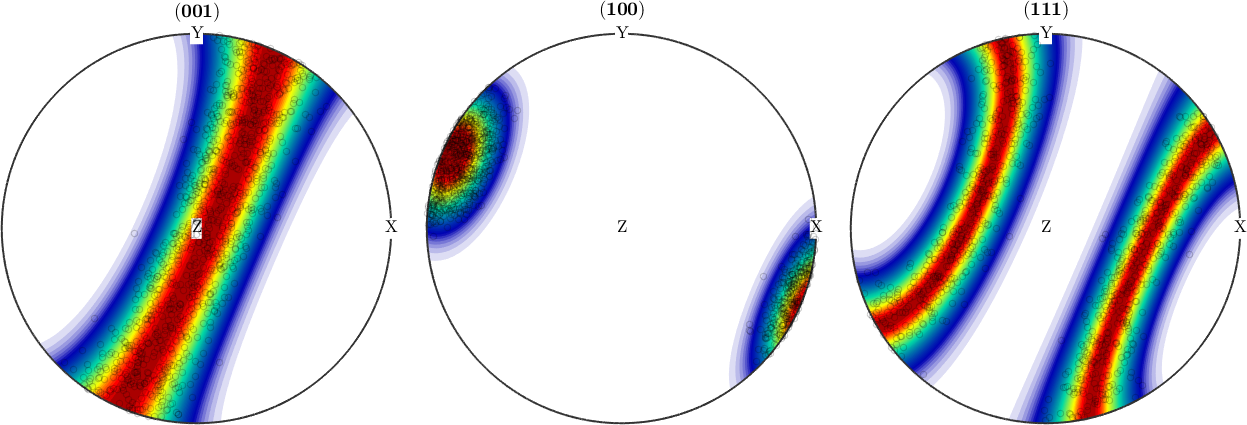From this simulated EBSD data, we can estimate the parameters of the Bingham distribution,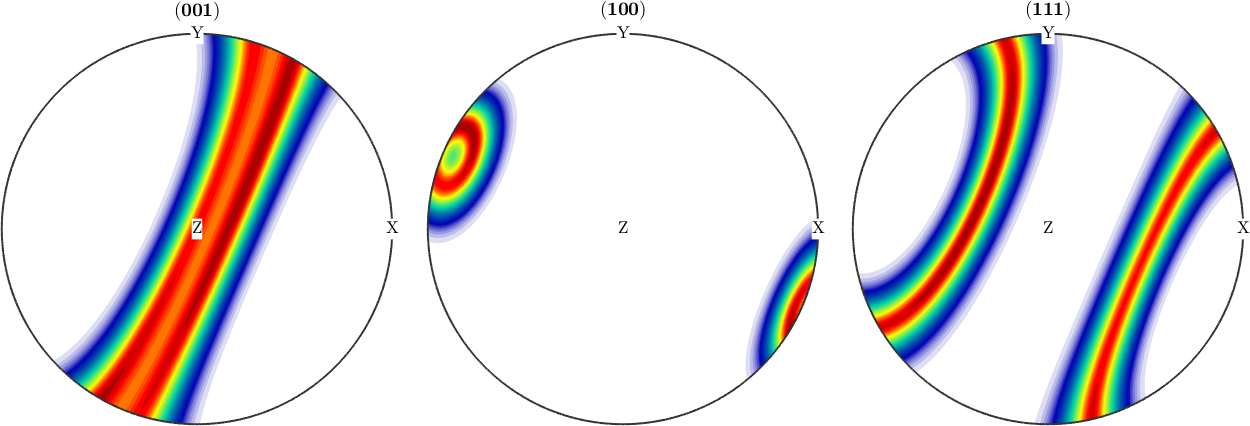## TODO

where U is the orthogonal matrix of eigenvectors of the orientation tensor and kappa the shape parameters associated with the U.

next, we test the different cases of the distribution on rejection

The spherical test case failed to reject for some level of significance, hence we would dismiss the hypothesis prolate and oblate.

## Prolate case and fibre distribution

The prolate case correspondes to a fibre.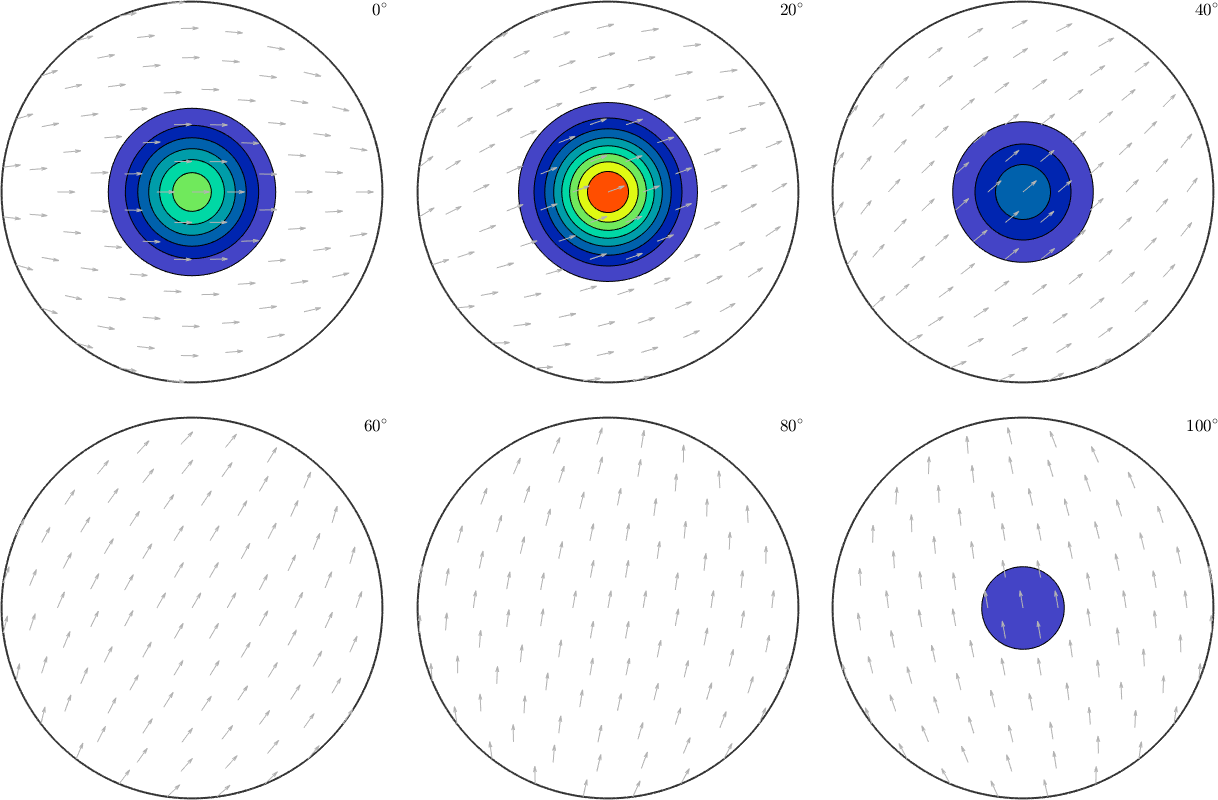As before, we generate some random orientations from a model odf. The shape in an axis/angle scatter plot reminds of a cigar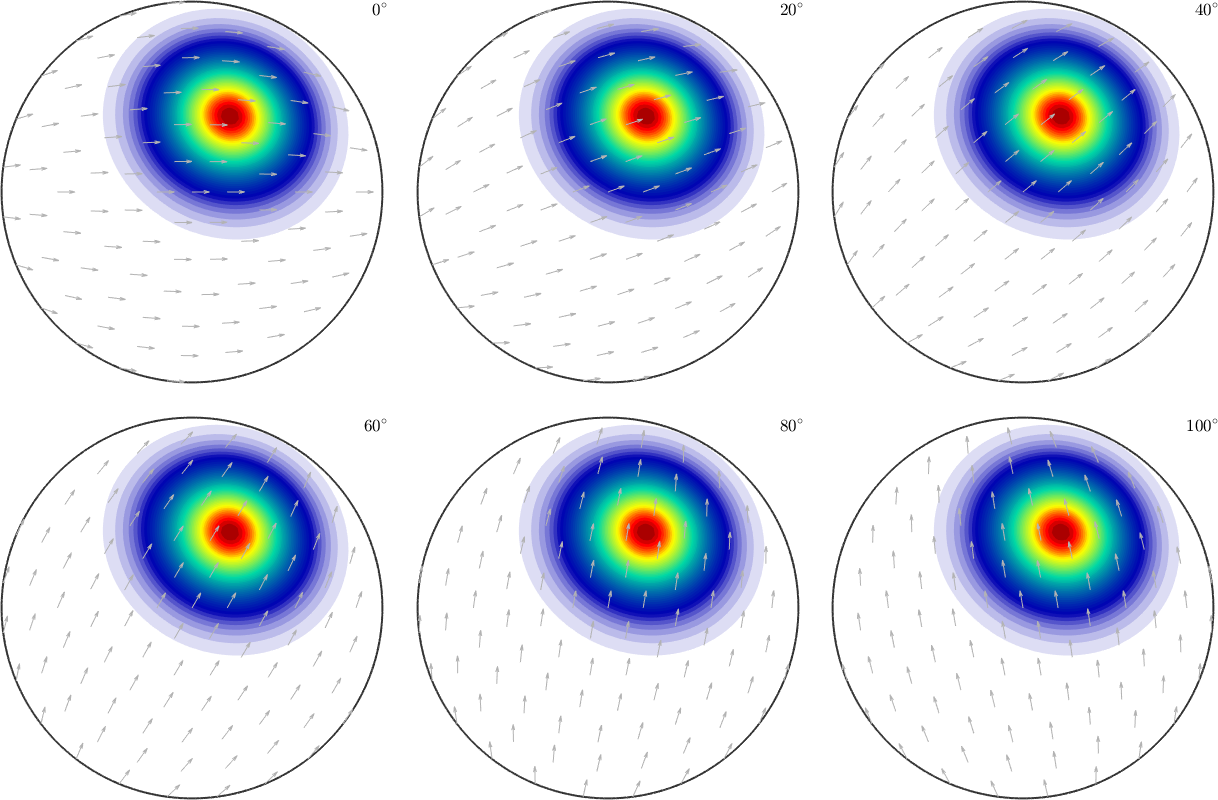We estimate the parameters of the Bingham distribution

and test on the three cases

The test clearly rejects the spherical and prolate case, but not the prolate. We construct the Bingham distribution from the parameters, it might show some skewness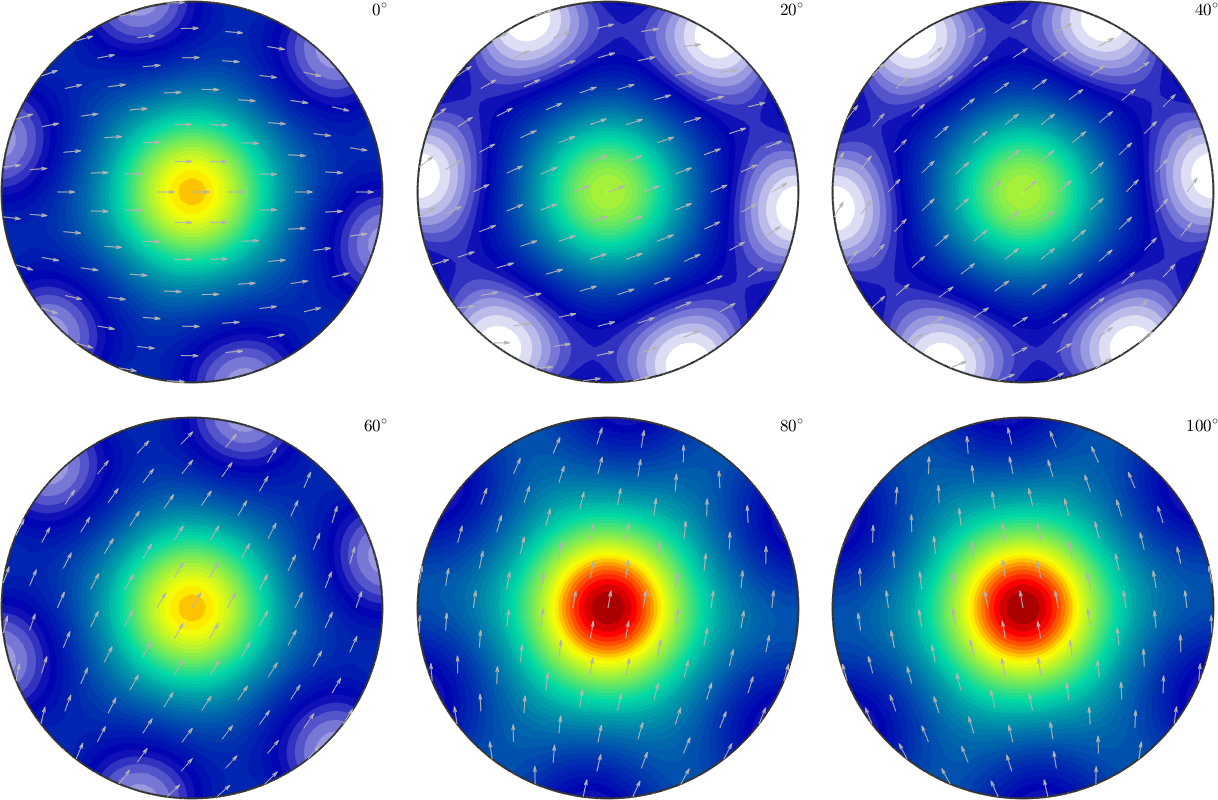## Oblate case

The oblate case of the Bingham distribution has no direct counterpart in terms of texture components, thus we can construct it straightforwardThe oblate cases in axis/angle space remind on a diskWe estimate the parameters again

and do the tests

the spherical and oblate case are clearly rejected, the prolate case failed to reject for some level of significanceBingham unimodal ODFBingham fibre ODFBingham spherical ODF